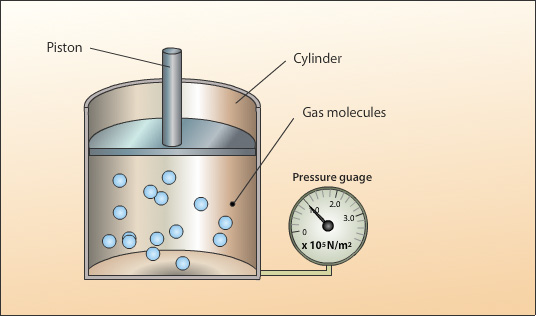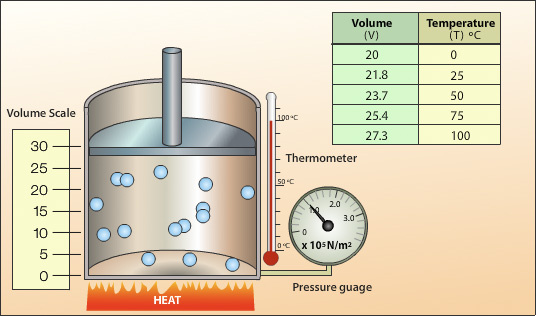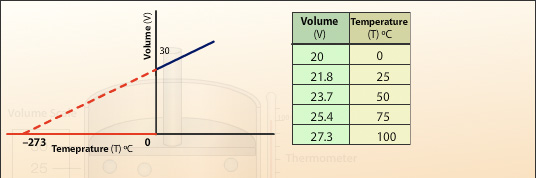## Charles' law

The relationship between the volume and temperature of a gas was first put forward by the French scientist Jacques-Alexandre-César Charles at around 1787 and is known as Charles’ Law.

Charles’ law states:

"For a fixed mass of gas, at a constant pressure, the volume (V) is directly proportional to the absolute temperature (T)."

Volume α Temperature

 Volume = constant Temperature

The animation below gives and explanation of Charles' law:A sealed cylinder with no leaks contains a fixed mass. In order to keep the gas pressure constant the piston is allowed to move freely so that the internal pressure created by the gas particles can equal the constant external pressure. If the internal pressure increases the piston will move up to allow the pressure to equalise.The above set up is used to investigate the relationship between temperature and volume for a gas. Heat energy is applied to the cylinder and the temperature of the gas increases. The average velocity of the gas particles increases resulting in an increase in the rate of collisions and the average force per collision. This produces an increase in pressure inside the cylinder, the cylinder pressure becomes greater than the external pressure and the piston moves up increasing the volume.By plotting the recorded values of volume (V) against temperature (T) a straight line is produced. We can see from the values that the gas expands uniformly with temperature.  We can extrapolate the straight line and see the relationship between cooling the gas and the volume. Further extrapolation gives the temperature at which the volume of gas would become zero. This temperature is at -273°C and is called the absolute zero of temperature.Converting the recorded temperatures into the Kelvin scale and plotting the volume (V) against the absolute temperature (T) gives a straight line which when extrapolated passes through the origin. This shows the volume of the gas is directly proportional to the absolute temperature of the gas. Doubling the temperature will double the volume. The gradient of the slope is the constant in Charles’ Law.

## Charles’ Law Example:

Using the example of the sealed cylinder above, the volume of gas at the start is recorded as 30 cm3 with a temperature of 30°C. The cylinder is heated further till the thermometer records 60°C. What is the volume of gas?

### Solution:

We know

V/T = constant

therefore,

V1/T1 = V2/T2

V1 = 30 cm3
T1 = 30°C = 30+273 = 303K (remember to convert from Celsius to Kelvin)
T2 = 60°C = 60+273 = 333K
V2  =
?

V1/T1 = V2/T2

 V2 = V1x T2 T1

 V2 = 30 x 333 303

= 32.97 cm3